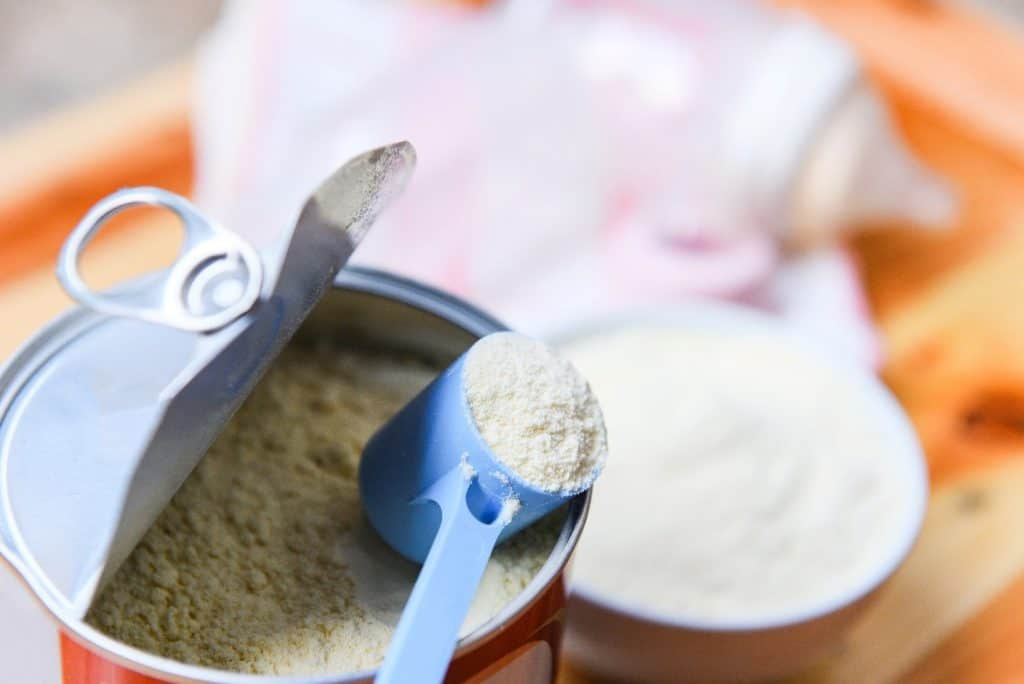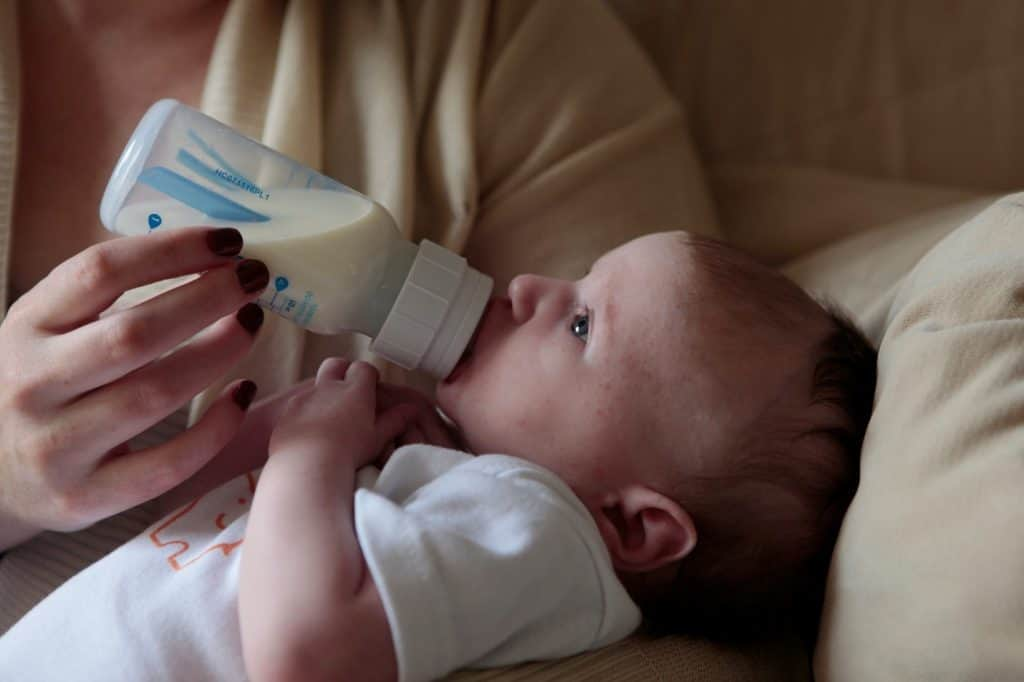# How Many Cans of Formula Per Month? 4 Factors to Consider

Being a parent means being prepared for everything your baby will ever need. If you are bottle-feeding your baby, that means you would want to be stocked with the right amount of baby formula. However, how many cans of formula per month should you have?

The number of cans of formula per month needed highly depends on factors such as the type of formula, the age of the baby, how many times the baby is fed, and how much milk the baby drinks per feeding.

For example, a newborn baby would require around 400 oz of formula in the first month. If a can of baby formula can prepare up to 200 oz of milk, then 2 cans are needed. However, if a smaller can of baby formula can only prepare up to 100 oz of milk, then 4 cans of baby formula are needed.

This article will discuss the several factors to consider determining how many cans of formula per month you should have at home.

1. ### Type of FormulaThere is a reason why the formula aisle at the grocery store is completely packed: there are a lot of different types of formulas to choose from. One of the challenges of being a parent is having to find the best formula for your baby.

Baby formulas can be categorized by their protein content. Most baby formula brands use cow’s milk protein as most babies do well with it. However, other options are available for babies who have difficulty with cow’s milk protein. Alternatives include soymilk protein and hydrolyzed protein formulas.

Soymilk protein formula is excellent for babies who are unable to process the lactose present in cow’s milk. For babies with even more severe sensitivities, there are formula products with all their proteins completely hydrolyzed.

However, when it comes to how many cans of formula per month parents should prepare, parents should consider the form in which the baby formula comes in as well.

There are three main forms of baby formula: powdered formula, concentrated liquid formula, and ready-to-use formula.

Perhaps the most commonly found type in the market is powdered formula. This is baby formula that comes in powder form and has to be mixed with water before feeding. Among the three choices, it is the most popular as it is the cheapest option.

Just like powdered formula, concentrated liquid formula has to be mixed with water for preparation as well. It is considered to be less messy to prepare compared to powdered formula, and concentrated liquid formula is considered to be commercially sterile, making it a good option for premature babies.

Lastly, ready-to-use formula is the most convenient of all forms of formula. No preparation is required as it can be fed to the baby directly. Like concentrated liquid formula, it is also commercially sterile. However, ready-to-use formula is unsurprisingly the most expensive option.

Aside from the different types and forms of formula, these products also come in varying sizes. All of these factors taken together will need to be considered when determining how much formula you would need in a month.

While you can get baby formula in larger packs or cans, it might be better to get multiple smaller cans. This way, an opened can does not last too long, thereby preventing unnecessary spoilage and contamination.

1. ### AgeUnderstandably, age is one of the major factors to consider when determining how many cans of formula per month you would need to prepare. As a growing baby continues to develop, you can expect their feeding schedules to change along the way.

The American Academy of Pediatrics outlines a feeding schedule for the first year of the baby. The feeding schedule differs for formula-fed babies and breastfed babies.

Newborns should be fed about 2 oz every 3-4 hours. This increases to 3-4 oz every 3-4 hours for 1-month-olds. Two- to 4-month-olds are fed 3-6 oz about 5-8 times a day. This changes to 4-6 oz about 4-6 times a day for 4- to 6-month-olds.

At 6-8 months, the feeding schedule changes to 6-8 oz for 3-5 times a day. At 8-12 months, this changes to 7-8 oz for 3-4 times a day. Babies a year old and older should then be receiving 16-24 oz per day.

Here is a table that summarizes the feeding schedule for formula-fed babies according to the American Academy of Pediatrics.

 Age Formula Fed Full-term Newborn 2 oz every 3-4 hours 1 Month 3-4 oz every 3-4 hours 2-4 Months 3-6 oz, 5-7 times per day 4-6 Months 4-6 oz, 4-6 times per day 6-8 Months 6-8 oz, 3-5 times per day 8-12 Months 7-8 oz, 3-4 times per day 1 Year and Older 16-24 oz per day

The summary table should give you a good idea as to how many cans of formula per month you would need. For example, if a full-term newborn needs 2 oz every 3-4 hours, that will roughly equate to 411 oz per month.

Knowing that number, you can then assess how many cans you would need depending on how much formula can be prepared from a single can. If a can of formula can prepare 200 oz of milk, then two cans of formula should suffice for the first month of the newborn.

Another example would be for a 5-month-old. According to the table, a 5-month-old should be fed 4-6 oz 4-6 times per day. That adds up to an estimate of around 750 oz per month. If a can of formula can prepare 200 oz of milk, then you would need 4 cans of formula for that month.

Understand that these sample calculations assume that a can of formula can prepare up to 200 oz of milk. However, you would be able to find other cans of formula that could be either bigger or smaller. Thus, it is important to check how much milk you can prepare from a single can of formula.

1. ### Number of FeedingsAs stated in the summary table above, babies can be fed with a range of number of feedings per day. It stands to reason that the number of feedings should be an important factor when considering how many cans of formula per month you should have since more feedings would require more formula.

Let’s take a look at the example of the 5-month-old again. The computation above was made with the average values. Since 5-month-olds should be getting 4-6 oz 4-6 times a day, the computation of 750 oz per month was made with the assumption of feeding the baby 5 oz for 5 times a day.

That value would be drastically different if the baby was being fed 4 times a day or 6 times a day. If the baby was being fed 5 oz 4 times a day, that would come to a total of 600 oz per month. On the other hand, feeding the baby 5 oz for 6 times a day would then add up to 900 oz per month.

Continuing with the assumption of a single can of formula that can prepare 200 oz of milk, feeding the 5-month-old 4 times a day would only need 3 cans of milk while feeding the same baby 6 times a day would need 5 cans of formula that month.

1. ### Amount Per FeedingsJust as the number of feedings significantly affects how many cans of formula per month are needed when taking care of a baby, so does the amount of formula being fed per feeding.

The case of the 5-month-old will be used as an example once more. Assuming that the baby is fed an average of 5 times per day, the total amount of formula needed per month will greatly depend on whether the baby takes 4 oz per feeding or if they take 6 oz per feeding.

If the baby gets 4 oz per feeding (for 5 feedings per day), that will come to a total of 600 oz per month. On the other hand, a baby getting 6 oz of formula per feeding at the same frequency would add up to a total of 900 oz per month.

With the assumption of a single can of formula capable of preparing 200 oz of milk, feeding the baby 4 oz per feeding would require 3 cans of formula that month while feeding the baby 6 oz of formula per feeding would require 5 cans of formula.

## Final Thoughts

When it comes to determining how many cans of formula per month are needed, there are a few factors to consider. The factors listed above include the type of formula, the baby’s age, how often the baby is being fed, and how much the baby is being fed per feeding.

To answer how many cans of formula per month are needed, it would really depend on the size of the can (i.e., how much milk can be prepared from a single can of formula).

With the wide variety of formula products available in the market, it is highly recommended to compute the total amount of formula needed in a month – instead of thinking of the number of cans. Once you have a total amount computed, you can then decide how many cans of baby formula are needed based on how much milk a single can of formula can prepare.